## 用“事实”说话 陆风X8车主用车报告

2015年05月28日 14:48 来源：www.chextx.com 超过：次关注

五一前后，陆风汽车正在举办盛大的优惠“FUN价季”活动，陆风X5全系最高优惠1万元，最低8.98万元起售，对持币待购的消费者是一大利好，对已经是准车主的用户则是一声叹息，“我买价未降，价降我已买，只恨降价迟，只怨买车早”，其实买车和炒股一样，永远买不到最低价，只要价位合理就可以出手了，而陆风X8各4S店也有数千元不等的优惠，且内饰已全面升级，对于需要大空间，喜欢越野，常往返城乡间的用户，此时购买是个不错的时机。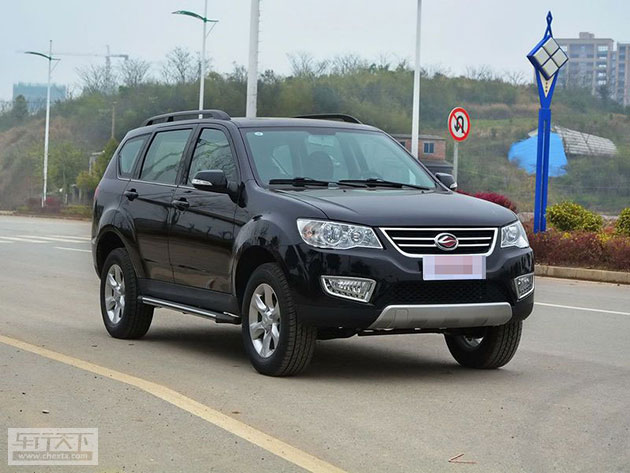2015款陆风X8增加了日间行车灯和车身侧裙边造型，车尾增加了镀铬装饰的“LAND WIND”字样，车顶的天线被换成了更加时尚的鲨鱼鳍天线造型。内饰中控台标配了8英寸液晶屏，具备了倒车可视、GPRS、蓝牙、胎压检测等众多功能，方向盘也由之前的三幅变成了四幅式设计，变更了手刹造型。新款内饰已旧貌换新颜，而“内力”依旧如往昔般出色，让我们以事实为根据、以数据为准绳，看看两位车主的用车报告。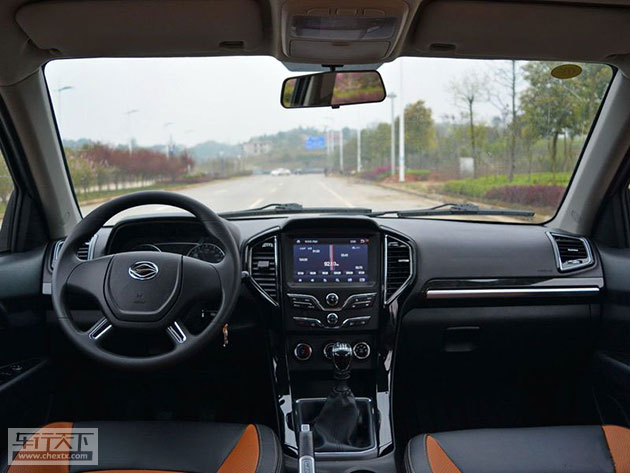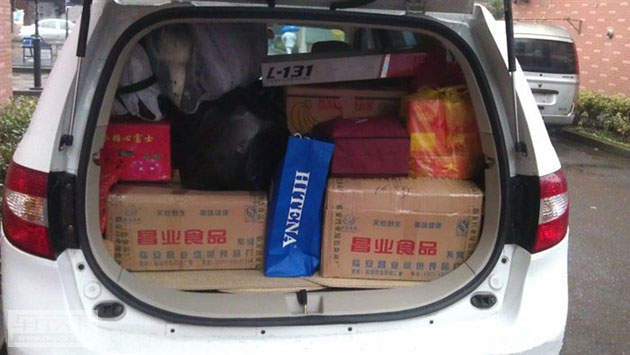李先生在杭州工作，老家在360公里外的山区，没买车前，每年春节回家和父母团聚的时候，因为没有直达车，要倒腾4趟车到家，每倒腾一趟车要排上数小时的队，在县城错过时间就没有车了，必须包车回去，常被黄鱼车宰上一刀。买了陆风X8以后，不再拎着大包小包的挤车了，今年回家一车4人，满满一车年货，X8的大空间优势得到体现，它拥有4636mm*1865mm*1810mm的车身尺寸，轴距达到2760mm，如果后排座椅放倒，后备箱容积可扩展到2460L, 可与MPV媲美。在同级车中不可多得。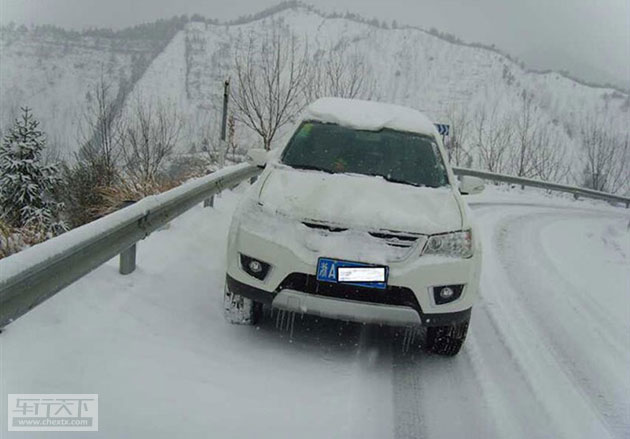在浙西山路遇到大雪，温度-5度，看保险杠下挂满了冰凌你就知道温度有多低了，山坡其实很陡，走路都要脚指头垫起来走，附近的景物可以当做参照物。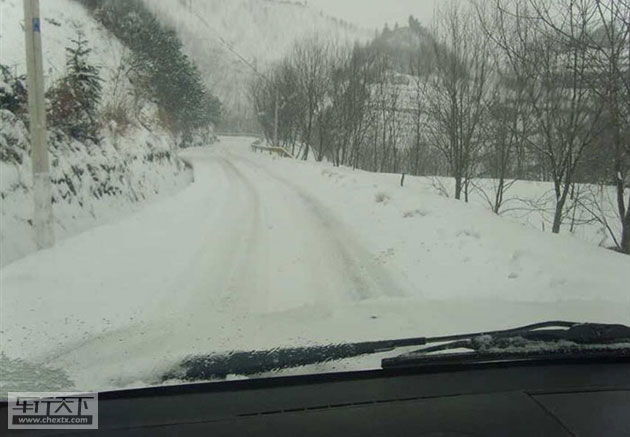大雪深度12厘米左右，雪下面是坚硬的冰，李先生的车没有防滑链，X8两驱表现不错，有伊顿差速锁的帮助已经很有信心对付这样的路面了。它确保车子在陷车或车轮打滑转时，能够安全制动，从容进退，使汽车在行驶过程中更加平稳有力。当然如果换成X8四驱，可以比两驱车开的更快，行驶更稳定一些。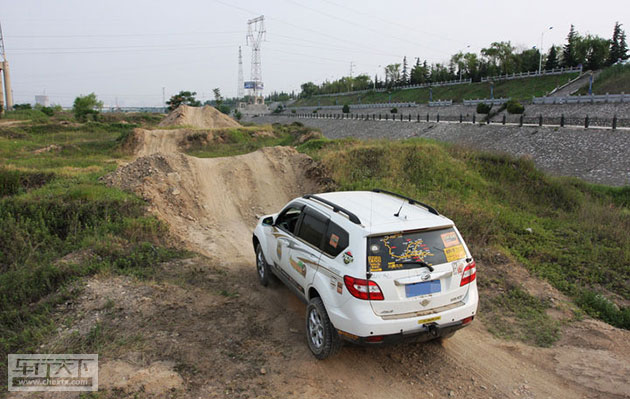如果李先生走的还算是寻常路，那么这位纯属是为“虐”车而来，这里是大秦独立团的兄弟们撒欢的风水宝地，面对连续的驼峰路面，那些普通城市SUV的小妖们速速退去吧！让我X8来！很多城市SUV依然采用的是承载式车身。这样的车体结构刚性并不足以支持越野，很容易在越野过程中产生变形，从而带来不必要的麻烦甚至影响安全。而陆风X8则采用了2纵11横、一体化贯穿性焊接车架结构，属于非承载式车身，其优点是有独立的大梁，底盘强度较高，车身刚性高，抗颠簸性能好；此外四个车轮受力再不均匀，也是由车架承担，而不会传递到车身上去。这样的车体结构足以支持陆风X8翻山越岭而依旧面不改色。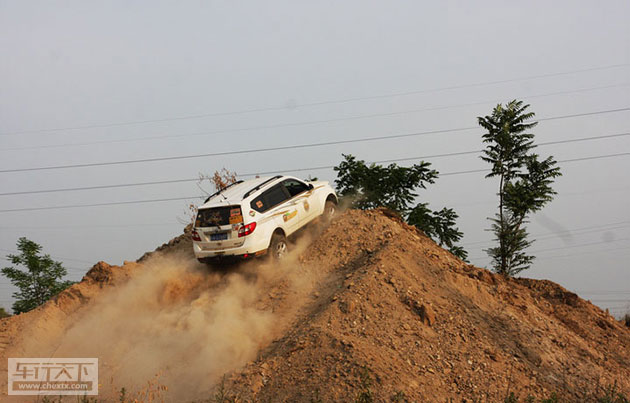一鼓作气势如虎， 黄沙弥漫气如虹。陆风X8最小离地间隙为200mm，接近角为27.4度，离去角为24.2度，有了傲人的资本，才敢将驼峰路，炮弹坑无情的践踏在脚下，才敢和你如此任性的玩耍。

所以，由此可以看出，无论是日常生活还是极限越野，陆风X8都可以满足你的要求，而且陆风X8现已进行改款，内饰的更新、配置的丰富，使得此款产品更加全面，拥有它你将不会后悔。

#### 相关文章

﻿
• 快速找车
• 选择品牌
• 选择品牌
• A  奥迪
• A  阿斯顿·马丁
• A  阿尔法·罗密欧
• B  宝沃
• B  布加迪
• B  巴博斯
• B  保时捷
• B  宾利
• B  奔驰
• B  宝马
• B  本田
• B  别克
• B  标致
• B  比亚迪
• B  宝骏
• B  北汽制造
• B  北汽新能源
• B  北汽幻速
• B  北汽威旺
• B  北京汽车
• B  奔腾
• B  北汽绅宝
• B  北汽昌河
• C  长安欧尚
• C  长安
• C  长安凯程
• C  长城
• D  大众
• D  道奇
• D  DS
• D  东南
• D  东风风神
• D  东风风行
• D  东风小康
• D  东风风度
• D  东风
• F  福特
• F  丰田
• F  菲亚特
• F  法拉利
• F  福田
• F  福迪
• F  福汽启腾
• G  观致
• G  广汽传祺
• G  广汽吉奥
• G  GMC
• H  红旗
• H  汉腾汽车
• H  哈弗
• H  哈飞
• H  海格
• H  海马
• H  华颂
• H  黄海
• H  华泰
• H  恒天
• J  捷途
• J  几何汽车
• J  捷达
• J  吉利汽车
• J  捷豹
• J  Jeep
• J  江淮
• J  江铃
• J  金杯
• J  九龙
• J  金旅
• K  凯翼
• K  凯迪拉克
• K  克莱斯勒
• K  科尼塞克
• K  卡威
• K  开瑞
• L  路虎
• L  林肯
• L  劳斯莱斯
• L  兰博基尼
• L  雷克萨斯
• L  铃木
• L  领克
• L  雷诺
• L  理念
• L  力帆
• L  莲花汽车
• L  猎豹
• L  路特斯
• L  陆风
• M  马自达
• M  MG
• M  MINI
• M  玛莎拉蒂
• M  摩根
• M  迈凯轮
• N  纳智捷
• O  欧拉
• O  欧宝
• O  讴歌
• O  欧朗
• Q  奇瑞
• Q  起亚
• Q  启辰
• R  日产
• R  荣威
• R  瑞麒
• S  SERES赛力斯
• S  三菱
• S  斯威汽车
• S  萨博
• S  smart
• S  斯柯达
• S  斯巴鲁
• S  思铭
• S  双龙
• S  上汽大通
• S  双环
• T  特斯拉
• T  腾势
• W  蔚来
• W  沃尔沃
• W  WEY
• W  五菱汽车
• W  五十铃
• W  威兹曼
• W  威麟
• X  现代
• X  雪佛兰
• X  星途
• X  雪铁龙
• X  小鹏汽车
• X  西雅特
• Y  一汽
• Y  英菲尼迪
• Y  英致
• Y  依维柯
• Y  野马汽车
• Y  永源
• Z  众泰
• Z  中华
• Z  中兴
• Z  知豆
• 选择车系
• 选择车系
• 车型对比
• 选择品牌
• 选择品牌
• A  奥迪
• A  阿斯顿·马丁
• A  阿尔法·罗密欧
• B  宝沃
• B  布加迪
• B  巴博斯
• B  保时捷
• B  宾利
• B  奔驰
• B  宝马
• B  本田
• B  别克
• B  标致
• B  比亚迪
• B  宝骏
• B  北汽制造
• B  北汽新能源
• B  北汽幻速
• B  北汽威旺
• B  北京汽车
• B  奔腾
• B  北汽绅宝
• B  北汽昌河
• C  长安欧尚
• C  长安
• C  长安凯程
• C  长城
• D  大众
• D  道奇
• D  DS
• D  东南
• D  东风风神
• D  东风风行
• D  东风小康
• D  东风风度
• D  东风
• F  福特
• F  丰田
• F  菲亚特
• F  法拉利
• F  福田
• F  福迪
• F  福汽启腾
• G  观致
• G  广汽传祺
• G  广汽吉奥
• G  GMC
• H  红旗
• H  汉腾汽车
• H  哈弗
• H  哈飞
• H  海格
• H  海马
• H  华颂
• H  黄海
• H  华泰
• H  恒天
• J  捷途
• J  几何汽车
• J  捷达
• J  吉利汽车
• J  捷豹
• J  Jeep
• J  江淮
• J  江铃
• J  金杯
• J  九龙
• J  金旅
• K  凯翼
• K  凯迪拉克
• K  克莱斯勒
• K  科尼塞克
• K  卡威
• K  开瑞
• L  路虎
• L  林肯
• L  劳斯莱斯
• L  兰博基尼
• L  雷克萨斯
• L  铃木
• L  领克
• L  雷诺
• L  理念
• L  力帆
• L  莲花汽车
• L  猎豹
• L  路特斯
• L  陆风
• M  马自达
• M  MG
• M  MINI
• M  玛莎拉蒂
• M  摩根
• M  迈凯轮
• N  纳智捷
• O  欧拉
• O  欧宝
• O  讴歌
• O  欧朗
• Q  奇瑞
• Q  起亚
• Q  启辰
• R  日产
• R  荣威
• R  瑞麒
• S  SERES赛力斯
• S  三菱
• S  斯威汽车
• S  萨博
• S  smart
• S  斯柯达
• S  斯巴鲁
• S  思铭
• S  双龙
• S  上汽大通
• S  双环
• T  特斯拉
• T  腾势
• W  蔚来
• W  沃尔沃
• W  WEY
• W  五菱汽车
• W  五十铃
• W  威兹曼
• W  威麟
• X  现代
• X  雪佛兰
• X  星途
• X  雪铁龙
• X  小鹏汽车
• X  西雅特
• Y  一汽
• Y  英菲尼迪
• Y  英致
• Y  依维柯
• Y  野马汽车
• Y  永源
• Z  众泰
• Z  中华
• Z  中兴
• Z  知豆
• 选择车系
• 选择车系
• 选择车型
• 选择车型
• 意见反馈